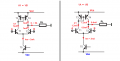# current mirror, double the gain

#### ntetlow

Joined Jul 12, 2019
63
I have been confused (not for the first time) about several of the points made in the article. Particularly where it says that the current mirror "doubles the gain of the differential amplifier" from Q8 collector to Q3 base.
I get that the current mirror duplicates the gain from Q1 but struggle to see that it doubles it.
Can some kind person enlighten me here?

#### Jony130

Joined Feb 17, 2009
5,456

#### ntetlow

Joined Jul 12, 2019
63
Without the current mirror. Q1 current mirror can swing from 0A to Iee (Iee is RE tail current). But by adding the current mirror we can "increase" the gain because now the load current can swing 2Iee.
Look at this schematic
So for the same change in the input voltage, the current is two times larger.
Thanks for the reply. I've recreated the circuit in Ltspice. The two inputs are in the same phase, I thought they should be 180 degrees out, so it seems both transistors would be on at the same time,

#### ntetlow

Joined Jul 12, 2019
63
Thanks for the reply. I've recreated the circuit in Ltspice. The two inputs are in the same phase, I thought they should be 180 degrees out, so it seems both transistors would be on at the same time,

#### Attachments

• 4.6 KB Views: 3

#### Jony130

Joined Feb 17, 2009
5,456
I thought they should be 180 degrees out,
No, why?
so it seems both transistors would be on at the same time,
As the name suggests the differential amplifier "reacts" to a voltage difference between two bases.

$\frac{I_{E1}}{I_{E2}} = e^{\frac{V_{BE1}- V_{BE2}}{26mV}}$

Without the input signal due to negative feedback we have Vbe1 = Vbe2 , thus have Ic1 = 0.5mA and Ic2 = 0.5mA.
For the positive signal at the Q1 base, we have Vbe1 > Vbe2. Therefore Ic1 increases, and Ic2 decreases by the same amount.
For example, if Ic1 rise from 0.5mA to 0.7mA at the same time the Ic2 current must decreases from 0.5mA to 0.3mA.
In short, we can say that a diff amp "steers" the tail current between one side (Q1) or to the other side (Q2). Depending on the voltage difference between the two bases.

From the small-signal point of view, we can also see how the current mirror increases the gain.
When VA = VB we have Vbe = Vbe2 thus, Ic1 = Ic2 = Iee/2 = 1mA
But if the input signal caused to Ic1 current to increase by 0.2mA (VA > VB). The load current will increase two times this value (2 * 0.2mA = 0.4mA ) due to the current mirror action.
Thus, the gain will double compared to the diff amp without the current mirror.http://www.ecircuitcenter.com/Circuits_Audio_Amp/Diff_Amp_w_Mirror/Diff_Amp_w_Mirror.htm

#### Attachments

• 8.4 KB Views: 2
Last edited:

#### eetech00

Joined Jun 8, 2013
3,421
Thanks for the reply. I've recreated the circuit in Ltspice. The two inputs are in the same phase, I thought they should be 180 degrees out, so it seems both transistors would be on at the same time,
A suggestion...study how current mirrors work.
In a basic current mirror, current from the diode connected transistor will be reflected (and limited) into the second transistor.
For the opamp, if the tail current is provide thru a resistor, the current is split between the two collectors of the PNP load transistors, so each transistor will receive 1/2 the total current thru the resistor. In addition, the current will be affected by a change to the supply voltage level. If the tail resistor is replaced with a current mirror, then each collector will potentially get the same current (well..almost the same current, but very close to the same) limited by the current mirror. Additionally, the current thru the mirror will be much less affected by a change to the supply voltage level.